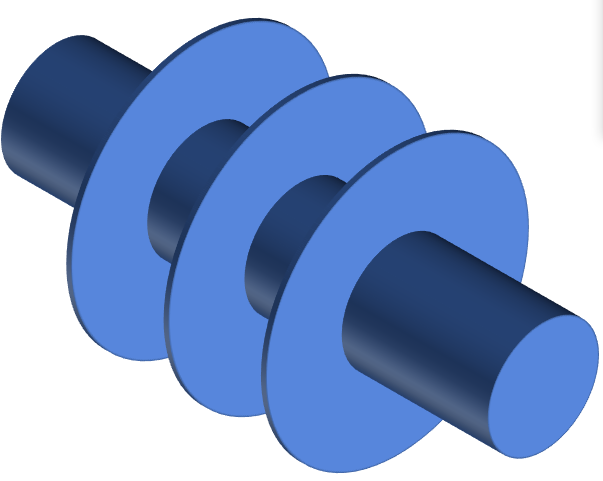# An electronic device with a temperature of 90°C (shown in

An electronic device with a temperature of 90°C (shown in golden colour) is embedded inside an aluminium heat exchanger with three fins (shown in blue) to enhance the convective heat transfer with the surrounding air. Calculate the amount of heat loss through the heat exchanger. The diameter of the electronic device is 10 cm while the total length of the device is 100 cm. The wall thickness of the heat exchanger surrounding the electronic device is 5 mm. The dimeter of the fins is 30 cm. Ignore the thickness of the fins. Estimate the fins as vertical planes. The ambient temperature is 20°C.

1. Drawing the thermal resistance circuit
2. Deriving the energy balance of the system
3. Finding the thermos-physical properties of air and aluminium
4. Calculation of heat transfer coefficients across the cylinder and the fins
5. Calculation of heat lossGet this answer with Solutioninn Study